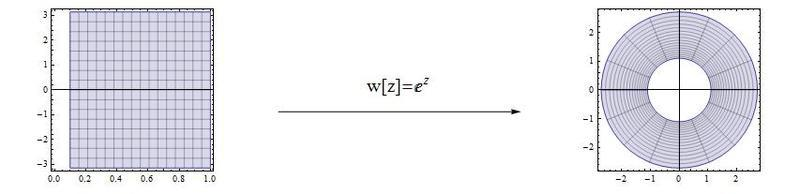# Plotting Complex functions in Mathematica

In a few of my books on Complex variables they show how you can look at a complex function as essentially a mapping from what plane to another.

Does anyone know if there would be a way to have mathematica plot how a complex function would transform one plane into the other?

Thanks for any help.

In a few of my books on Complex variables they show how you can look at a complex function as essentially a mapping from what plane to another.

Does anyone know if there would be a way to have mathematica plot how a complex function would transform one plane into the other?

Thanks for any help.

You can plot a parametric region. For example, the mapping $e^z\to w$ maps the square region in the z-plane to the washer region in the w-plane:

Code:
w[z_] := Exp[z];
p1 = ParametricPlot[{x, y}, {x, 1/10, 1}, {y, -Pi, Pi}, AspectRatio -> 1];
p2 = ParametricPlot[{Re[w[z]], Im[w[z]]} /. z -> x + I*y, {x, 1/10, 1}, {y, -Pi, Pi},
PlotRange -> All];
myarrow = Show[Graphics[{{Arrow[{{-0.5, 0}, {0.5, 0}}]},
Text[Style["w[z]=\!$$\*SuperscriptBox[\(E$$, $$z$$]\)", 20], {0, 0.1}]}]];
GraphicsGrid[{{p1, myarrow, p2}}]Ok, now modify my code to map the annulus $1\leq r\leq 5$ in the z-plane under the transformation $1/z\to w$ into the w-plane.